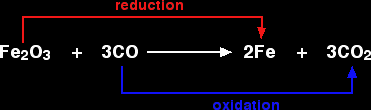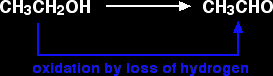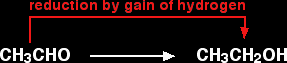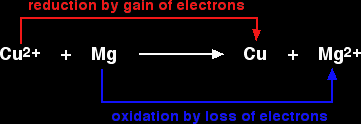# Redox reactions

This page looks at the various definitions of oxidation and reduction (redox) in terms of the transfer of oxygen, hydrogen and electrons. It also explains the terms oxidising agent and reducing agent.

### Oxidation and reduction in terms of oxygen transfer

Definitions

• Oxidation is gain of oxygen.
• Reduction is loss of oxygen.

For example, in the extraction of iron from its ore:Because both reduction and oxidation are going on side-by-side, this is known as a redox reaction.

#### Oxidising and reducing agents

An oxidising agent is substance which oxidises something else. In the above example, the iron(III) oxide is the oxidising agent.

A reducing agent reduces something else. In the equation, the carbon monoxide is the reducing agent.

• Oxidising agents give oxygen to another substance.
• Reducing agents remove oxygen from another substance.

### Oxidation and reduction in terms of hydrogen transfer

These are old definitions which aren't used very much nowadays. The most likely place you will come across them is in organic chemistry.

#### Definitions

• Oxidation is loss of hydrogen.
• Reduction is gain of hydrogen.

Notice that these are exactly the opposite of the oxygen definitions.

For example, ethanol can be oxidised to ethanal:You would need to use an oxidising agent to remove the hydrogen from the ethanol. A commonly used oxidising agent is potassium dichromate(VI) solution acidified with dilute sulphuric acid.

Ethanal can also be reduced back to ethanol again by adding hydrogen to it. A possible reducing agent is sodium tetrahydridoborate, NaBH4. Again the equation is too complicated to be worth bothering about at this point.#### An update on oxidising and reducing agents

• Oxidising agents give oxygen to another substance or remove hydrogen from it.
• Reducing agents remove oxygen from another substance or give hydrogen to it.

### Oxidation and reduction in terms of electron transfer

This is easily the most important use of the terms oxidation and reduction.

#### Definitions

• Oxidation is loss of electrons.
• Reduction is gain of electrons.

It is essential that you remember these definitions. There is a very easy way to do this. As long as you remember that you are talking about electron transfer:

# RIG

Oxidation Is Loss Reduction Is Gain

#### A simple example

The equation shows a simple redox reaction which can obviously be described in terms of oxygen transfer.

CuO + Mg → Cu + MgO

Copper(II) oxide and magnesium oxide are both ionic. The metals obviously aren't. If you rewrite this as an ionic equation, it turns out that the oxide ions are spectator ions and you are left with:#### A last comment on oxidising and reducing agents

If you look at the equation above, the magnesium is reducing the copper(II) ions by giving them electrons to neutralise the charge. Magnesium is a reducing agent.

Looking at it the other way round, the copper(II) ions are removing electrons from the magnesium to create the magnesium ions. The copper(II) ions are acting as an oxidising agent.

##### Warning!

This is potentially very confusing if you try to learn both what oxidation and reduction mean in terms of electron transfer, and also learn definitions of oxidising and reducing agents in the same terms.

It is recommend that you work it out if you need it. The argument (going on inside your head) would go like this if you wanted to know, for example, what an oxidising agent did in terms of electrons:

• An oxidising agent oxidises something else.
• Oxidation is loss of electrons (OIL RIG).
• That means that an oxidising agent takes electrons from that other substance.
• So an oxidising agent must gain electrons.

Or you could think it out like this:

• An oxidising agent oxidises something else.
• That means that the oxidising agent must be being reduced.
• Reduction is gain of electrons (OIL RIG).
• So an oxidising agent must gain electrons.

Understanding is a lot safer than thoughtless learning!